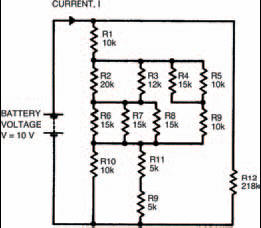# More and more complex circuitsElectronics guide > On the boards > More and more complex circuits

Circuits we’ve looked at so far have been very simple, really. Much more complex ones await us over the coming chapters. We’ll also be introduced to several new components, such as capacitors, diodes, transistors, and ICs. Fortunately, most of the new circuits and components follow these basic laws we’ve studied, so you’ll be able to understand their operation without much ado.

The aim of these basic laws is to simplify complex circuits so that we may understand them and how they work, with reference to the circuits we have already seen. For example, the circuit in Figure 2.9 is quite complex. It consists of many resistors in a network. But by grouping the resistors into smaller circuits of only series and parallel resistors, it’s possible to simplify the whole circuit into one overall resistance.

In fact, I’m going to leave you with that problem now. What you have to do, is to calculate the current, I, which flows from the battery. You can only do that by first finding the circuit’s overall resistance. If you can’t do it by calculation you can always do it by building the circuit up on breadboard and measuring it. Resistor and multi-meter tolerances, of course, will mean the measured result may not give exactly the same result as the calculated one.Figure 2.9 A comparatively complex circuit showing a number of resistors in parallel and in series. By breaking the circuit down into groups, the overall resistance can be calculated

Meanwhile, there’s always the quiz over the page to keep you occupied!

<< The next circuit